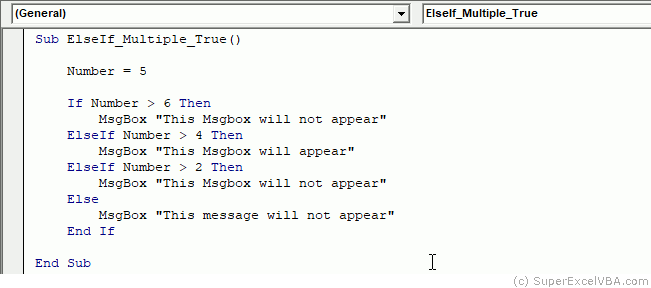# VBA If...Else

The If...Then works as a conditional for VBA: it performs an action (codes) only if the condition (shortly after If) is equal to True (or result in True).

    Sub conditional()
If True then 'If [Condition] Then (in this example the condition is True)
Msgbox "This Msgbox should appear"
End If

If False then
Msgbox "This Msgbox should not appear"
End If
End Sub


The If function works properly only for conditions that result in True or False.

Note that the statement to be executed is between the If and the End If.

    'If Condition Then
'   Codes
'   ...
'End If


The condition may also come from a variable.

    condition_check = True

If condition_check then
Msgbox "This Msgbox should appear"
End If


To run a statement while the If condition is False, use Else.

    condition_check = False

If condition_check then
Msgbox "This Msgbox should not appear" 'Because condition_check is False
Else 'However, because condition_check is False, the code below will be executed
Msgbox "This Msgbox should appear"
End If


You can use the Comparison Operators to create conditions.

    Number = 4

If 3 <= Number then 'This will return True
Msgbox "This Msgbox should appear"
Else
Msgbox "This Msgbox should not appear"
End If


Through the interaction with the user, one can create smart executions:

Sub If_Example()

Answer = Inputbox("How much is 10 - 3?")

Msgbox "You are correct!"
Else
Msgbox "You are wrong"
End If

End Sub


Note that the equal sign = used in If is a comparison operator. In this case, it does not assign a value to a variable but verifies if the condition was met.

## ElseIf

You can use ElseIf if you need to include more statements within the same If function.

Sub ElseIf_Example()

Dim Score As Single

Msgbox "Congratulations, you got it all right!"
Msgbox "You passed the test"
Msgbox "You did not pass the test"
Else
Msgbox "Please enter a valid score"
End If

End Sub


### Multiples True in ElseIf

When using ElseIf it is possible that multiple conditions result in True. In this case only the first, in order of execution (from top to bottom) will be executed, and the others will not.

Sub ElseIf_Multiple_True()

Number=5

If Number > 6 Then
Msgbox "This Msgbox will not appear"
ElseIf Number > 4 Then
Msgbox "This Msgbox will appear"
ElseIf Number > 2 Then
Msgbox "This Msgbox will not appear"
Else
Msgbox "This message will not appear"
End If

End Sub